# Engineering Mechanics July 2011

Unit-I

1. a) A sphere of weight 100N is tied to a smooth wall by a string as shown in Fig. 1. Find the tension T in the string and reaction R of the wall.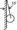Fig. 1

b) Find the moment of 100 N force acting at B about point A as shown in Fig. 2.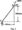c) The beam AB of span 12m. Shown in Fig. 3 is hinged at A and is on rollers at  B. Determine the reactions at A and B for the loading shown in Fig. 3. (8)

OR

Find the forces in all the members of the truss shown in Fig. 4. results. Fig.4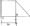Unit-II

2. a) Using principle of virtual work, determine for what range of force P;a block of weight 1000N, can be kept in equilibrium on an inclined plane as shown in Fig. 5.b) Determine the force P required to start the movement of the wedge as shown in Fig. 6. The angle of friction for all surfaces of contact is 15°.Fig. 6

OR

a)  The ladder shown in Fig. 7 is 6m long and is supported by a horizontal floor and vertical wall. The coefficient of friction between the floor and the ladder is 0.25 and between wall and ladder is 0.4. The weight of the ladder is 200N and may be considered as concentrated at G. The ladder also supports a vertical load of at C which is at a distance of lm from B. Determine the least value of ‘a‘ at which the ladder may be placed without slipping. Determine the reaction at that stage.(16)Fig. 7

Unit-III

3.  a) A load of 20KN is to be lifted by a differential wheel and axle. It consists of differential axle of 250mm and 300mm diameter and the wheel diameter is 800mm. Find the effort required, if the efficiency of the machine is 55%.

b) What force is required to raise the load W shown in Fig. 8. Assume the efficiency of the system to be 85%.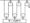Fig. 8

OR

a) Determine the moment of inertia of the section shown in Fig. 9 about an axis passing through the centroid and parallel to the top most fibre of the section.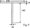Unit-IV

4.  a) An elevator cage of a mine shaft weighing 8KN, when empty, is lifted or lowered by means of a wire rope. Once a man weighing 600N, entered it and lowered with uniform acceleration such that when a distance of 187.5m was covered, the velocity of the cage was 25m/sec. Determine the tension in the rope and the force exerted by the man on the floor of the cage.

b)  A person wants to jump over a ditch as shown in Fig. 10. Find the minimum velocity with which he should jump.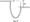OR

a) In the system of connected blocks shown in Fig. 11, A under A and C is 0.2. Find the tension in the string and the acceleration of each block. The pulleys are supposed to be weightless and frictionless.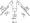Unit-V

5. A wagon weighing 500KN starts from rest, runs 30 m down one percent grade and strikes the bumper post. If the rolling resistance of the track is 5N/kN, find using work, energy principle; the velocity of the wagon, when it strikes the post. If the bumper spring which compresses 1mm for every 15kN; determine by how much this spring will be compressed.

OR

a)  A gun weighing 300KN fires a 5KN projectile with a velocity of 300 m/sec. With what velocity will the gun recoil? If the recoil is overcome by an average force of600KN, how far will the gun travel? How long will it take?

b) A 80N body moving to the right at a speed of 3m/sec. strikes a 10 N body, that is moving to the left at a speed of lOm/sec. The final velocity of ION body is 4m/sec. to the right. Calculate the coefficient of restitution and the final velocity of the SON body.